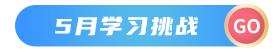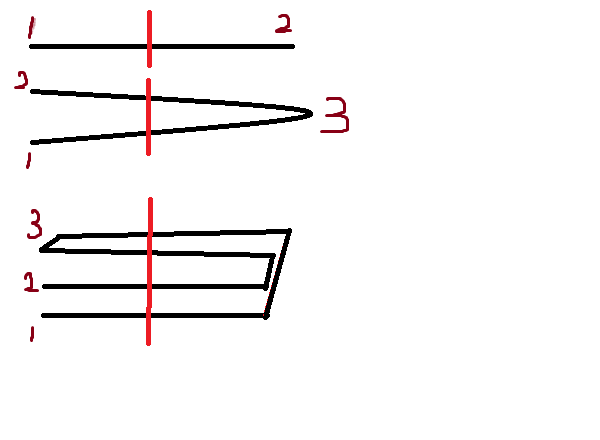如果先对折1次，中间切一刀，可以得到3根面条。

那么，连续对折10次，中间切一刀，会得到多少面条呢？

1、还是那句话，先数学分析，再动手。规律题，不妨多画几步，让规律呈现在眼前。0         2

1         2+2^0

2         2+2^0+2^1

3         2+2^0+2^1+2^2

...       ...

Code:

``````#include <stdio.h>

int main()
{
int i,t,sum;
t=1,sum=2;
for(i=0;i<10;i++)
{
sum+=t;
t=t*2;
}

printf("%d\n",sum);

return 0;
}``````